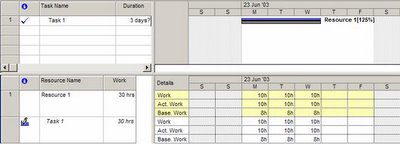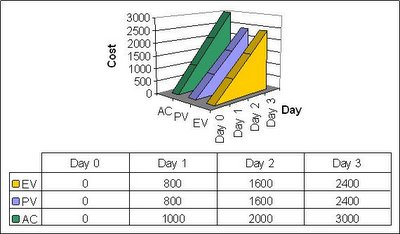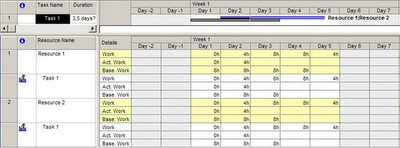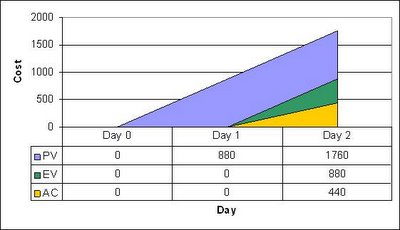# Introduction to EVM – The Cake## 14 Aug Introduction to EVM – The Cake

Never wonder why each week the project is always on budget but the project always finishes costing more or finishing latter?

Imagine that you have a three-day project with one task done by one resource. You plan accordingly and at day 1 you plan 8 hours, at day 2 you plan another 8 and at day 3 another 8.

On day 1 the resource reports 10 hours. That’s great you are ahead of schedule! The other day it reports another 10 hours. At this time you are ecstatic you have done the project and saved half a day (life could not be better). At day 3 the resource reports another 10 hours. Wow… What happened? You go talk to your team member and he tells you that the project was sub estimated and the work instead of taking 8 hours to finish each day it took 10. This is bad news. How could you have seen this coming? Always busy with so many projects and the accounting numbers never reflect what’s happening on the field.

This happens when analysing only the accounting variance (work planned minus work performed). However, there is a better way.

The Earned Value technique is broadly used as a performance measurement method for projects of any size. It enables a project manager to assess if there are variances from the base project plan that require some sort of corrective action.

This method presents an overview of the health of the schedule, cost and scope of a project and reflects the actual performance of the project. This is accomplished by calculating three main values for each task on the project plan:

1. Planned Value (PV): also known as Budgeted Cost of Work Scheduled (BCWS) is the value of the cost (calculated at the initial planned cost) of the work planned to have been done so far;

2. Actual Cost (AC): also known as Actual Cost of Work Performed (ACWP) is the value of the cost (calculated at the actual cost) of the work done so far;
3. Earned Value (EV): also known as Budgeted Cost of Work Performed (BCWP) is the value of the cost (calculated at the initial planned cost) of the work actually done so far. Some people like to think of it as the “physical progress of the work”.

The following figure illustrates the concepts based on our three days project:Let us assume that our resource costs 100/h. The grey bar represents the initial plan. The key values are:

1. PV at day 1 is 100*8; at day 2 it’s equal to PV at day 1 plus 100*8; at day 3 it’s equal to PV at day 3 plus 100*8;

2. AC at day 1 is 100*10; at day 2 it’s equal to AC at day 1 plus 100*10; at day 3 it’s equal to AC at day 2 plus 100*10;

3. EV at day 1 is 100*8; at day 2 it’s equal at EV at day 1 plus 100*8; at day 3 it’s equal to EV at day 2 plus 100*8.

The figure bellow displays in a graph the three key EV values:So lets see what would be our conclusions each day. Since AC is greater than EV this would tell us that our resource is doing more work to get the same results. At day 2 that situation maintains and at day 3 the same result. So you could have act since day 1 to try to solve the situation. Another curious conclusion is that if we look closer at the results EV and PV are always the same. This tell us that we are actually on schedule and the project is going to finish at the planned date since the work actually done is always the same as the work planned. Great, now we can get a clear picture of the current project status instead of just hopping for the best.

Lets look at one more example.Resource 1 costs 100€/h while Resource 2 costs 10€/h. As we can see, we are currently one day late and the current date is Day 3. Instead of starting working at Day 1 the resources only started at Day 2 and reported only 4 hours each instead of 8. The figure bellow displays in a graph the three key EV values:So PV at day 1 is equal 100*8+10*8. PV at day 2 is equal to PV at day 1 plus 100*8+10*8. EV for day 1 is zero since no work was done. At day 2 EV is EV at day 1 plus 100*8+10*8. AC at day 1 is zero, at day 2 is AC at day 1 plus 100*4+10*4.

These values represent two things:

1. Since the work actually done (EV) is greater at day 2 than the work reported (AC) then we are more productive than expected;Since the work planned (PV) at day 2 is greater than the work reported as finished (EV) then we are behind schedule.

2. Since the work planned (PV) at day 2 is greater than the work reported as finished (EV) then we are behind schedule.

Next week article: Introduction to EVM – The Frosting.

J